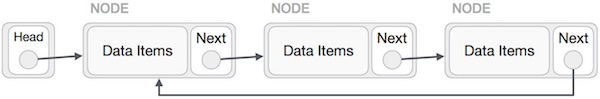# Data Structure - Circular Linked List

Circular Linked List is a variation of Linked list in which the first element points to the last element and the last element points to the first element. Both Singly Linked List and Doubly Linked List can be made into a circular linked list.

## Singly Linked List as Circular

In singly linked list, the next pointer of the last node points to the first node.## Doubly Linked List as Circular

In doubly linked list, the next pointer of the last node points to the first node and the previous pointer of the first node points to the last node making the circular in both directions.As per the above illustration, following are the important points to be considered.

• The last link's next points to the first link of the list in both cases of singly as well as doubly linked list.

• The first link's previous points to the last of the list in case of doubly linked list.

## Basic Operations

Following are the important operations supported by a circular list.

• insert − Inserts an element at the start of the list.

• delete − Deletes an element from the start of the list.

• display − Displays the list.

## Insertion Operation

Following code demonstrates the insertion operation in a circular linked list based on single linked list.

### Example

```insertFirst(data):
Begin
create a new node
node -> data := data
if the list is empty, then
else
while next of temp is not head, do
temp := next of temp
done
next of temp := node
end if
End
```

## Deletion Operation

Following code demonstrates the deletion operation in a circular linked list based on single linked list.

```deleteFirst():
Begin
it is Underflow and return
else
while next of ptr is not head, do
ptr := next of ptr
next of ptr = next of head
end if
End
```

## Display List Operation

Following code demonstrates the display list operation in a circular linked list.

```display():
Begin
Nothing to print and return
else